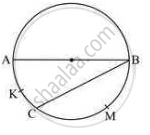# In the Following Figure, Seg Ab is a Diameter of the Circle, M (Arc Akc) = 40° . Find the Value of M (Arc Bmc). - Geometry

In the following figure, seg AB is a diameter of the circle, m (arc AKC) = 40°. Find the value of m (arc BMC).#### Solution

Seg AB is a diameter

M (arc ACB) =180° [Angle Made in Semi circle = 180°]

M (arc AKC) +m (arc BMC) = m (arc ACB) [Arc Addition

Property]

40+ m (arc BMC) = 180°

M (arc BMC) = 180° -40°

M (arc BMC) =140°

Concept: Tangent to a Circle
Is there an error in this question or solution?